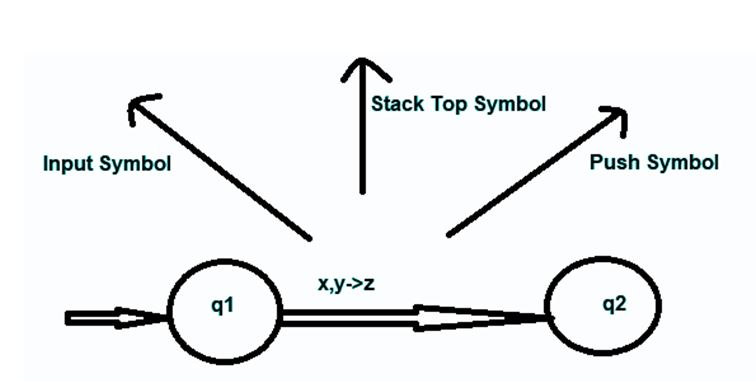# Pushdown Automaton (PDA) | Theory of Computation

Here, we are going to learn about the pushdown automatic (PDA) which is a kind of automation in theory of computation.
Submitted by Hrithik Chandra Prasad, on July 20, 2019

Pushdown Automaton (PDA) is a kind of Automaton which comes under the theory of Computation that appoints stack. The word Pushdown stands due to the fact that the stack can be pushed down as operations can only work on the elements which are on the top of the stack. A PDA can store an infinite amount of information. It is used to identify Context-free languages.

"Pushdown Automation" = "Finite State Machine" + "Stack"

A finite state machine does not employ any stack and only bothers about the input signal and the current state that does not work in the case of context free grammar. Push Down Automata is different from finite state machine because,

1. It uses top of the stack for deciding which transition is to be taken.
2. While performing the transition, it can handle or manipulate the stack.

Pushdown Automaton (PDA) reads the provided string from left to right direction. The current state, input symbol and the symbol at TOS (Top of the Stack) are being indexed in the table and helps in choosing a transition, this happens at each step. While performing a transition, PDA can manipulate stack in two ways, either it can push a symbol at the top of the stack or can pop out a symbol from the stack.

Formal definition of PDA,

PDA can be betokened formally by a 7-tuple (Q, ∑, S, δ, q0, I, F) where,

1. Q is the number of states. It is finite.
2. is an input alphabet. It is a finite set.
3. S stands for stack symbols.(which can be pushed and popped from the stack).
4. δ is the transition function which is Q × (∑ ∪ {ε}) × S × Q × S*. It is a finite subset.
5. q0 is the start or initial or beginning state (q0 ∈ Q).
6. I is the initial stack top symbol (I ∈ S).
7. F is the set of accepting states (F ∈ Q).

In a specified state, PDA will read the symbol which is at the top of the stack and the input signal and move to a new state after changing the symbol of the stack.

Consider the following diagram which demonstrates transition in a PDA, from State q1 to state q2 described as x, y->z.In the above scenario, you can observe that if the current state of machine is q1, The input symbol is 'x' and 'y' is at the Top of Stack symbol then we can carry out push and pop operation by popping 'y' and pushing 'z' on the top of the stack and can proceed to state q2.

Some important points of PDA:

• A PDA is used to check whether a given grammar is context free grammar or not.
• A grammar is accepted if it reaches the end state on using of all its input symbols.
• There are some other notations of the PDA that are used. They are:
1. Instantaneous Description: For a PDA, instantaneous description is given by,
2. ```    triplet (q,w,s), where
q is the current state of the machine
w is the set of input symbols that are remaining
s is the stack.
```
3. Turnstile Notation: It defines the moves of PDA based on ID notation. Transition is defined as '⊢'

Preparation

What's New

Top Interview Coding Problems/Challenges!